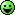# ₦airaland Forum

Welcome, Guest: Join Nairaland / LOGIN! / Trending / Recent / New
Stats: 2,294,411 members, 5,039,553 topics. Date: Tuesday, 16 July 2019 at 09:17 AM

## If You Can't Solve This As A Math Teacher, Hope You Wil Learn A Lesson(mr Shape) - Education - Nairaland

 If You Can't Solve This As A Math Teacher, Hope You Wil Learn A Lesson(mr Shape) by Mrshape: 12:14pm On May 05 Maths tutors/lecturers or professors have to be patient with does who are willing to learn, You are their teacher because you learnt it first not because you are more intelligent.Let's try this out I will bring solution tomorrow.HmmmEverywhere Green.Re: If You Can't Solve This As A Math Teacher, Hope You Wil Learn A Lesson(mr Shape) by Mrshape: 12:21pm On May 05 Show workings Re: If You Can't Solve This As A Math Teacher, Hope You Wil Learn A Lesson(mr Shape) by Mrshape: 1:36pm On May 05 Anyone? Re: If You Can't Solve This As A Math Teacher, Hope You Wil Learn A Lesson(mr Shape) by femi4: 10:09pm On May 05 EA = AD = xDiagonal = semicircle diameter = √(x²+x²) = x√2Radius of semicircle = x√2/2Now AF² = x² -(x√2/2)²AF² = x² - 2x²/4 = x²/2AF = x/√2But AF + FG = 14√2 x/√2 + x√2/2 = 14√2 2x +2x = 4*14 x = 14R of semi circle = x√2/2 = 7√2r of the circle = 7√2/2Area of the green region = Area of the semicircle - Area of the circleπ (7√2)²/2 - π (7√2/2)²π(98/2 - 98/4) = π 98/4 = 22/7 * 98/4 = 77 units² Re: If You Can't Solve This As A Math Teacher, Hope You Wil Learn A Lesson(mr Shape) by Martinez39: 10:54pm On May 05 femi4:EA = AD = xDiagonal = semicircle diameter = √(x²+x²) = x√2Radius of semicircle = x√2/2Now AF² = x² -(x√2/2)²AF² = x² - 2x²/4 = x²/2AF = x/√2But AF + FG = 14√2 x/√2 + x√2/2 = 14√2 2x +2x = 4*14 x = 14R of semi circle = x√2/2 = 7√2r of the circle = 7√2/2Area of the green region = Area of the semicircle - Area of the circleπ (7√2)²/2 - π (7√2/2)²π(98/2 - 98/4) = π 98/4 = 22/7 * 98/4 = 77 units² You are correct. I got the same thing too. Re: If You Can't Solve This As A Math Teacher, Hope You Wil Learn A Lesson(mr Shape) by Mrshape: 6:36pm On May 06 femi4:EA = AD = xDiagonal = semicircle diameter = √(x²+x²) = x√2Radius of semicircle = x√2/2Now AF² = x² -(x√2/2)²AF² = x² - 2x²/4 = x²/2AF = x/√2But AF + FG = 14√2 x/√2 + x√2/2 = 14√2 2x +2x = 4*14 x = 14R of semi circle = x√2/2 = 7√2r of the circle = 7√2/2Area of the green region = Area of the semicircle - Area of the circleπ (7√2)²/2 - π (7√2/2)²π(98/2 - 98/4) = π 98/4 = 22/7 * 98/4 = 77 units²Good one sir √√√√√√√√√√√(11/10) Re: If You Can't Solve This As A Math Teacher, Hope You Wil Learn A Lesson(mr Shape) by naturalwaves: 5:27pm On Jun 12 Mrshape:Good one sir √√√√√√√√√√√(11/10)I bet you did not realise that there are a lot of badazz mathematicians on Nairaland.Re: If You Can't Solve This As A Math Teacher, Hope You Wil Learn A Lesson(mr Shape) by Mrshape: 5:30pm On Jun 12 naturalwaves:I bet you did not realise that there are a lot of badazz mathematicians on Nairaland.I have been fetching them out lately with my post sir,It gives me joy to see like minds sir. Re: If You Can't Solve This As A Math Teacher, Hope You Wil Learn A Lesson(mr Shape) by naturalwaves: 5:33pm On Jun 12 Mrshape:I have been fetching them out lately with my post sir,It gives me joy to see like minds sir.Lolz.They are much o. I do not know the names you have on your list already. Perhaps I could add more. Re: If You Can't Solve This As A Math Teacher, Hope You Wil Learn A Lesson(mr Shape) by Mrshape: 5:43pm On Jun 12 naturalwaves:Lolz.They are much o. I do not know the names you have on your list already. Perhaps I could add more.Also check ifada123 thats my main account, go through my post you will see more of them.I have a lot of them following me.